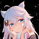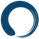# Quadratic Least Squares Moving Average - Smoothing + Forecast

1237 views
1237
Introduction

Technical analysis make often uses of classical statistical procedures, one of them being regression analysis, and since fitting polynomial functions that minimize the sum of squares can be achieved with the use of the mean, variance, covariance...etc, technical analyst only needed to replace the mean in all those calculations with a moving average, we then end up with a low lag filter called least squares moving average ( lsma ).

The least squares moving average could be classified as a rolling linear regression , altho this sound really bad it is useful to understand the relationship of both methods, both have the same form, that is ax + b, where a and b are coefficients of the model. However in a simple linear regression a and b are constant, while the lsma use variables instead.

In a simple lsma we model the relationship of the closing price (dependent variable) with a linear sequence (independent variable), therefore x = 1,2,3,4..etc. However we can use polynomial of higher degrees to model such relationship, this is required if we want more reactivity. Therefore we can use a quadratic form, that is ax^2 + bx + c, where a,b and c are variables.

This is the quadratic least squares moving average (qlsma), a not so official term, but we'll stick with it because it still represent the aim of the filter quite well. In this indicator i make the calculations of the qlsma less troublesome, therefore one might understand how it would work, note that in general the coefficients of a polynomial regression model are found using matrix calculus.

The Indicator

A qlsma, unlike the classic lsma , will fit better to the price and will be more reactive, this is the advantage of using an higher degrees for its calculation, we can model more complex relationship.

lsma in green, qlsma in red, with both length = 200

However the over/under shoots are greater, i'll explain why in the next sections, but this is one of the drawbacks of using higher degrees.

The indicator allow to forecast future values, the ahead period of the forecast is determined by the forecast setting. The value for this setting should be lower than length, else the forecasts can easily over/under shoot which heavily damage the forecast. In order to get a view on how well the forecast is performing you can check the option "Show past predicted values".

Of course understanding the logic behind the forecast is important, in short regressions models best fit a certain curve to the data, this curve can be a line ( linear regression ), a parabola (quadratic regression) and so on, the type of curve is determined by the degree of the polynomial used, here 2, which is a parabola. Lets use a linear regression model as example :

ax + b where x is a linear sequence 1,2,3...and a/b are constants. Our goal is to find the values for a and b that minimize the sum of squares of the line with the dependent variable y, here the closing price, so our hypothesis is that :

closing price = ax + b + ε

where ε is white noise, a component that the model couldn't forecast. The forecast of the closing price 14 step ahead would be equal to :

closing price 14 step aheads = a(x+14) + b

Since x is a linear sequence we only need to sum it with the forecasting horizon period, the same is done here with :

a*(n+forecast)^2 + b*(n + forecast) + c

Note that the forecast proposed in the indicator is more for teaching purpose that anything else, this indicator can't possibly forecast future values, even on a meh rate.

Low lag filters have been used to provide noise free crosses with slow moving average, a bad practice in my opinion due to the ability low lag filters have to overshoot/undershoot, more interesting use cases might be to use the qlsma as input for other indicators.

On The Code

Some of you might know that i posted a "quadratic regression" indicator long ago, the original calculations was coming from a forum, but because the calculation was ugly as hell as well as extra inefficient (dogfood level) i had to do something about it, the name was also terribly misleading.

We can see in the code that we make heavy use of the variance and covariance, both estimated with :

VAR(x) = SMA (x^2) - SMA (x)^2

COV(x,y) = SMA (xy) - SMA (x) SMA (y)

Those elements are then combined, we can easily recognize the intercept element c, who don't change much from the classical lsma .

As Digital Filter

The frequency response of the qlsma is similar to the one of the lsma , those filters amplify certain frequencies in the passband, and have ripples in the stop band. There is something interesting about those filters, first using higher degrees allow to greater boost of the frequencies in the passband, which result in greater over/under shoots. Another funny thing is that the peak/valley of the ripples is equal the peak or valley in the ripples of another lsma of different degree.

The transient response of those filters, that is impulse response, step response...etc is related to the degree of the polynomial used, therefore lets denote a lsma of degree p : lsma(p), the impulse response of lsma (p) is a polynomial of degree p, and the step response is simple a polynomial of order p+1.

This is why it was more interesting to estimate the qlsma using convolution, however we can no longer forecast future values.

Conclusion

I proposed a more usable quadratic least squares moving average , with more options, as well as a cleaner and more efficient code. The process of shrinking the original code is made easier when you know about the estimations of both variance and covariance.

I hope the proposed indicator/calculation is useful.These LSMA are really impressive, but could a slightly less effective/reactive but non repainting version be possible?, particularly an adaptive one, the adaptive LSMA you already made is really impressive but uses barindex, the TStep is fairly adaptive, but it's a step filter vs smoothed moving average.
AntwortenCharlesVein7777
@CharlesVein7777, This indicator doe's not repaint, repainting using the bar_index element occur with specific operations that are not used here.
AntwortenCharlesVein7777
@CharlesVein7777, The Tstep LSMA might be repainting due to the use of a cumulative average, i'am working on a non repainting version. Other LSMA scripts won't repaint.
AntwortenThis is an amazing write up, really great work. Much appreciated :)
Antworten